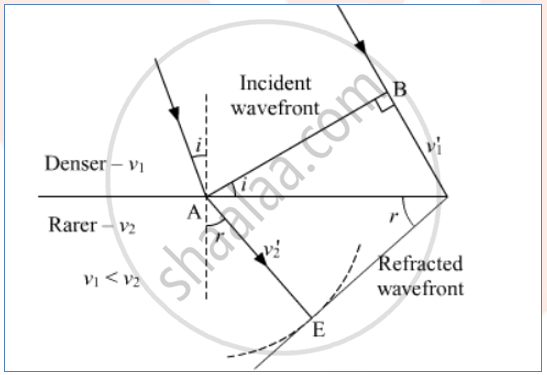# Define the Term, “Refractive Index” of a Medium. Verify Snell’S Law of Refraction When a Plane Wavefront is Propagating from a Denser to a Rarer Medium. Solution - Physics

#### Question

Define the term, “refractive index” of a medium. Verify Snell’s law of refraction when a plane wavefront is propagating from a denser to a rarer medium. Solution

#### Solution

The factor by which speed of light gets reduced with respect to the “speed of light in vacuum” is said to be the refractive index for the medium.

μ = c/ν or ν = c/mu

Here v is the speed of light in the medium, c is the speed of light in vacuum and μ is the defined refractive index for the medium.Concept: Snell’s Law
Is there an error in this question or solution?